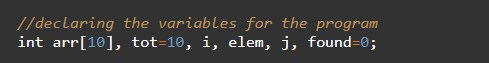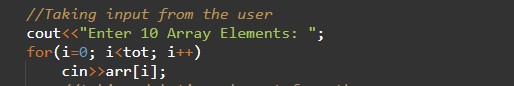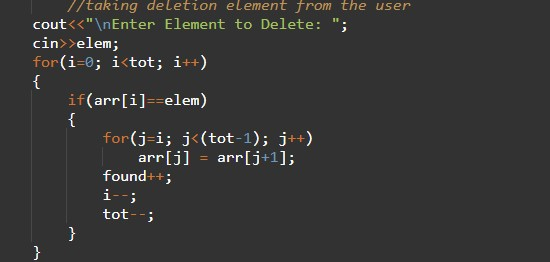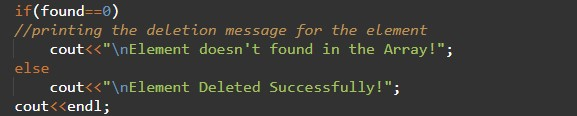# C++ Program to Delete Array Element

In this tutorial you will learn about the C++ Program to Delete Array Element and its application with practical example.

## C++ Program to Delete Array Element

In this tutorial, we will learn to create a C++ program that will delete the element of Array using C++ programming.

## Prerequisites

Before starting with this tutorial we assume that you are best aware of the following C++ programming topics:

• Operators in C++ Programming.
• Basic Input and Output function in C++ Programming.
• Basic C++ programming.
• For loop in C++ Programming.

## Program for Delete the element of Array:-

An array is a collection of similar data type elements. In today’s program, we will take an array in input from the user and then we will take the element for deleting from the array. After deleting the element from the array we will reprint the array.

## Program code:-

To Delete an element from the array

## Output:-In the above program, we have first initialized the required variable.• arr = it will hold the elements in an array.
• tot = it will hold the number of elements in an array.
• i = it will hold the integer value to control the array.
• Elem = it will hold the location for delete element size of the array.

Taking input from the user in an array of elements.Deleting the array element.Printing the Program CodeIn this tutorial we have learn about the C++ Program to Delete Array Element and its application with practical example. I hope you will like this tutorial.